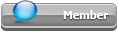## Featured Articles

Check out the latest featured articles.## File Library# Cp/cv Ratio

This topic has been archived. This means that you cannot reply to this topic.
7 replies to this topic
|

### #1DANA-IF

DANA-IF

Gold Member

•• Members
•• 160 posts

Posted 16 March 2016 - 05:43 AM

Hello all ,

I'm wondering please what we should call the Cp/Cv ratio for liquid  , it's called  gamma or k ratio ?

Thanks

### #2shantanuk100

shantanuk100

Gold Member

•• Members
•• 104 posts

Posted 16 March 2016 - 06:20 AM   Best Answer

Dear Dana,

Both Gamma and K are used to denote the Specific Heat Ratio.
The Specific Heat Ratio is usually denoted by Gamma by Chemical, Process, Aerospace Engineers.

The letter is usually used in place of Gamma by Mechanical Engineers, Thermal engineers and those more concerned with thermodynamics.

Regards,

Shantanu

Edited by shantanuk100, 17 March 2016 - 03:01 AM.

### #3latexman

latexman

Gold Member

•• ChE Plus Subscriber
•• 1,018 posts

Posted 16 March 2016 - 06:33 AM

Call it the "specific heat ratio".  Everyone who has the knowledge knows what that means.

### #4DANA-IF

DANA-IF

Gold Member

•• Members
•• 160 posts

Posted 16 March 2016 - 09:17 AM

Another question :

I'm wondering please if anyone know the difference between Cp/Cv (ent method ) and Cp/Cv (gamma) on hysys ?

Thanks a lot !

Regards !

### #5fallah

fallah

Gold Member

•• ChE Plus Subscriber
•• 4,684 posts

Posted 16 March 2016 - 11:58 AM

I'm wondering please what we should call the Cp/Cv ratio for liquid  , it's called  gamma or k ratio ?

Appears here a reality about the OP qestion has been forgotten...!

Contrary to gases in liquids, as is the subject of OP question, because are supposed to be incompressible Cp and Cv have almost same values hence only a single value of specific heat is applied for them; then the specific heat ratio would be almost not applicable...

### #6shantanuk100

shantanuk100

Gold Member

•• Members
•• 104 posts

Posted 17 March 2016 - 02:43 AM

Dear Dana,

1. I had failed to notice you mention liquid in the question. That is a very important point as Mr.Fallah has rightly pointed out.

When you consider gases, this ratio is called Gamma or K as mentioned earlier.

2. For liquids, the Cp and Cv become equal, so the ratio of Cp to Cv is always 1. So it doesn't have a specific name as such because it is always 1.

3. Let me illustrate, the Enthalpy of a system is defined as follows  ---  H = U + PV

So , for getting specific heat capacity at constant pressure we partially differentiate wrt to temperature (under a constant pressure process).

Partially differentiating the above equation (H = U + PV) at constant pressure, we get,

dH/dT = dU/dT + d(PV)/dT

dH/dT = dU/dT + PdV/dT + VdP/dT

Since it is a partial differential at constant pressure, we get the partial Del (P) = 0

And also since liquids are considered almost incompressible, we also get Del (V) = 0

So this gives,

dH/dT = dU/dT for incompressible fluids

Cp = Cv

4. If I were to explain it in physical terms, the change in enthalpy of a system is accounted for by corresponding change in the internal energy (U) of the system, and by the work being done (PdV Term), and by the VdP term.

But since Cp is defined at a constant pressure (dP=0) and since the volume change of incompressible fluids is too small for any considerable work to be done (dV=0), both those terms become null and your enthalpy change has to then only go into changing the internal energy change and nothing else. So, now, since the changes are equal, their respective ratios with temperature differentials will be the same thus making your Cp equal to Cv.

4. Now since your Cp and Cv become equal, their ratio is always = 1.

So for liquids, there still exists a specific heat ratio, but is usually always 1, so it doesn't make much sense to give it a name. It can still be called by Gamma or K, but even then, would always equal 1.
So no specific name is ever needed or used anywhere (Only in the case of liquids)

Cheers,

Shantanu

Edited by shantanuk100, 18 March 2016 - 12:44 AM.

### #7rohansharma01

rohansharma01

Brand New Member

•• Members
•• 1 posts

Posted 16 May 2016 - 06:14 AM

Hello,

I need to know temperature of natural gas after pressure reduction.

Input gas pressure is P1=70 Bar G and temperature is T1= 33 degree celsius and pressure after reduction is P2=25 Bar G.

The equation that I have come across is T2 /T1 = (P2/ P1)^(k-1)/k

On site the temperature after reduction is 11 degree celsius.

Can someone  help in how to obtain the desired result from above equation or is their any other equation.

### #8breizh

breizh

Gold Member

•• ChE Plus Subscriber
•• 4,640 posts

Posted 16 May 2016 - 06:23 AM

Hi ,

Make sure you are using the proper unit , Pressure in bar absolute and T in Kelvin , for cp/cv a value close to 1.3 should be good , I found 1.27 in litterature .

Probabaly good also to introduce the Z factor in the calculation .

P*V ^k=cte & P*V=Z*R*T

Note :

a)This should be an iterative calculation with assumption on Z2 .

I've added a calculator to calculate Z .

b)The composition of natural gas is also needed to calculate Z because it's a mixture.

http://checalc.com/s...aturalgasZ.html

Good luck

Breizh

Edited by breizh, 17 May 2016 - 01:05 AM.

### Similar Topics